# Introduction

Way back in 2013, DeepMind presented the Deep Q-Network (DQN) agent applied to Atari 2600 games. This agent grabbed screenshots from Atari games as input and used Q-learning to predict and take the best action. With traditional Q-learning, the entire state space must be represented in the Q-table, the table that stores state-action pairs with their Q-values. DQN uses a neural network as a function estimator to estimate this Q-fuction, rather than storing the Q-values explicitely. (Mnih et al., 2013)

Here, we'll implement a simplified version of the DQN agent applied to the Gym Lunar Lander environment. For this first implementation, rather than take screen grabs and use those to build our state, we'll use the state provided by Gym directly, removing that task to focus more explicitely on the algorithm itself. In a follow-up post, we'll drop the built in state and instead use game pixels to build the state.

Start by importing our libraries. We check if we're running in Google Colab to install additional libraries.

import gym
import torch
import torch.nn as nn
import torch.nn.functional as F
import pandas as pd
import random
import numpy as np
import matplotlib
import matplotlib.pyplot as plt
from itertools import count
from collections import deque, namedtuple
if COLAB:
!pip install box2d

is_ipython = 'inline' in matplotlib.get_backend()
if is_ipython:
from IPython import display


Set a seed for experiment reproducability.

SEED = 42


# Network Architecture

Our first step is to define our neural network. We'll use a stardard fully connected neural network with 4 hidden layers, each with 64 nodes except the last, which starts to trim down before the output layer. We use relus as our activation functions between layers.

class DQN(nn.Module):

def __init__(self, inputs, outputs):
super().__init__()

self.fc1 = nn.Linear(in_features=inputs, out_features=64)
self.fc2 = nn.Linear(in_features=64, out_features=64)
self.fc3 = nn.Linear(in_features=64, out_features=64)
self.fc4 = nn.Linear(in_features=64, out_features=32)
self.out = nn.Linear(in_features=32, out_features=outputs)

def forward(self, t):
t = F.relu(self.fc1(t))
t = F.relu(self.fc2(t))
t = F.relu(self.fc3(t))
t = F.relu(self.fc4(t))
t = self.out(t)
return t


# Experience Replay

When iterating through environments, we receive immediate feedback from the environment in the form of rewards. In a typical Q-learning algorithm, the Q-table is updated every iteration by calculating the Bellman equation for a given state-action pair. For our DQN agent, this won't work so well. We have two problems. One, since we receive rewards and want to make an update immediately, we only have a batch of one to calculate the gradient from before making our next move, and two, sequential actions and rewards are highly correlated. Concretely, the action and received reward of state $s_t$ is directly influenced by the action and reward received at state $s_{t-1}$.

To solve these two problems, we introduce a buffer to store experience tuples, defined as a tuple of a state, action, reward, and next state. For implementation reasons, we also store if the action resulted in a termination of the environment. The buffer of these tuples solves the batching problem for calculating gradients, but if we just take sequential experiences, we'll end up still having highly correlated samples. Instead, this is where Mnih, et al. introduced Replay Memory. When sampling from this buffer, we sample randomly rather than take the $n$ most recent samples. This solves our correlation issue, and keeps the buffer quite simple. (Mnih et al., 2013)

Experience = namedtuple("Experience", field_names=[
"state", "action", "reward", "next_state", "done"])

class ReplayMemory(object):
"""
https://pytorch.org/tutorials/intermediate/reinforcement_q_learning.html
"""

def __init__(self, buffer_size, batch_size, seed):
self.memory = deque(maxlen=buffer_size)
self.batch_size = batch_size
self.seed = random.seed(seed)

def push(self, state, action, reward, next_state, done):
self.memory.append(Experience(state, action, reward, next_state, done))

def sample(self, device):
"""
Sample a set memories.
Code adapted from a post from Chanseok Kang:
https://goodboychan.github.io/python/reinforcement_learning/pytorch/udacity/2021/05/07/DQN-LunarLander.html
"""
experiences = random.sample(self.memory, k=self.batch_size)

states = torch.from_numpy(
np.vstack([e.state for e in experiences if e is not None])).float().to(device)
actions = torch.from_numpy(
np.vstack([e.action for e in experiences if e is not None])).long().to(device)
rewards = torch.from_numpy(
np.vstack([e.reward for e in experiences if e is not None])).float().to(device)
next_states = torch.from_numpy(np.vstack(
[e.next_state for e in experiences if e is not None])).float().to(device)
dones = torch.from_numpy(np.vstack(
[e.done for e in experiences if e is not None]).astype(np.uint8)).float().to(device)

return (states, actions, rewards, next_states, dones)

def __len__(self):
return len(self.memory)


# The Agent

For illustration purposes, we'll define two agents, a simplified, single network agent, and a full, two network agent. The SimpleDQNAgent acts exactly as one might expect if you're familiar with Q-learning in general. We select actions by using an %\epsilon%-greedy policy, and when random actions are not taking we query the neural network for the best action given the state.

To update the q-fuction, input comes into the agent in %[s, a, r, s']% tuples, which it saves off to replay memory. If replay memory contains enough examples to batch, we'll performing a learning iteration. As described above, we sample randomly from replay memory for our minibatch, which we use to update the neural network. To calculate our loss, we use the Bellman equation to calculate the Q-values for all the states in our minibatch. To gather these, we take the rewards for each state, and add to that a Q-value calculated by inputing the %s'% to the neural network, representing future Q-value. This we multiply by %\gamma% before adding to rewards. Terminal states here are removed.

class SimpleDQNAgent():
def __init__(
self,
state_vector_length,
num_actions,
alpha=.001,
eps=1,
eps_decay=0.995,
eps_min=0.05,
gamma=0.9,
batch_size=64,
seed=None
):
self.num_actions = num_actions
self.eps = eps
self.eps_decay = eps_decay
self.eps_min = eps_min
self.gamma = gamma
self.device = torch.device("cuda" if torch.cuda.is_available() else "cpu")

self.step = 0
self.policy_net = DQN(state_vector_length, num_actions).to(self.device)
params=self.policy_net.parameters(), lr=alpha)

self.memory = ReplayMemory(100000, batch_size, seed)

if seed != None:
np.random.seed(seed)

def select_action(self, s):
self.step += 1
if np.random.random() < self.eps:
action = np.random.randint(0, self.num_actions)
else:
action = self._get_best_action(s)

return action

def _get_best_action(self, s):
action = self.policy_net(torch.tensor([s]).to(
self.device)).argmax(dim=1).to(self.device).item()
return action

def update_q(self, s, a, s_prime, r, done):
self.memory.push(s, a, r, s_prime, done)
self.step += 1

if done:
self.eps = max(self.eps_min, self.eps * self.eps_decay)

if len(self.memory) > self.memory.batch_size:
experiences = self.memory.sample(self.device)
self.learn(experiences)

def learn(self, experiences):
states, actions, rewards, next_states, dones = experiences

next_q_values = self.policy_net(
next_states).detach().max(1).unsqueeze(1)
q_targets = rewards + self.gamma * next_q_values * (1 - dones)
current_q_values = self.policy_net(states).gather(1, actions)

loss = F.mse_loss(current_q_values, q_targets)
loss.backward()
self.optimizer.step()

def save_network(self, outfile):
torch.save(self.policy_net.state_dict(), outfile)

self.policy_net.eval()


The full DQNAgent uses two networks rather than one. With a single network, we're constantly adjusting to a moving target. When the loss is propogated, we're calculating the values of the loss against values produced from the network itself. This causes the network to eat its own tail, so to speak. Instead in our full implementation we use two networks, a policy network and a target network. When selecting next actions and calculating current Q-values, we query the policy network. The target network, on the other hand, is used for calculating the next Q-values for backpropogating the loss to the policy network. We copy the paramaters of the policy network to the target network on some inverval, which we can define as a hyper-parameter.

class DQNAgent():

def __init__(
self,
state_vector_length,
num_actions,
alpha=.001,
eps=1,
eps_decay=0.995,
eps_min=0.05,
gamma=0.9,
batch_size=64,
seed=None
):
self.num_actions = num_actions
self.eps = eps
self.eps_decay = eps_decay
self.eps_min = eps_min
self.gamma = gamma
self.device = torch.device("cuda" if torch.cuda.is_available() else "cpu")

self.step = 0
self.policy_net = DQN(state_vector_length, num_actions).to(self.device)
self.target_net = DQN(state_vector_length, num_actions).to(self.device)
self.target_net.eval()
params=self.policy_net.parameters(), lr=alpha)

self.memory = ReplayMemory(100000, batch_size, seed)

if seed != None:
np.random.seed(seed)

def select_action(self, s):
self.step += 1
if np.random.random() < self.eps:
action = np.random.randint(0, self.num_actions)
else:
action = self._get_best_action(s)

return action

def _get_best_action(self, s):
action = self.policy_net(torch.tensor([s]).to(
self.device)).argmax(dim=1).to(self.device).item()
return action

def update_q(self, s, a, s_prime, r, done):
self.memory.push(s, a, r, s_prime, done)
self.step += 1

if done:
self.eps = max(self.eps_min, self.eps * self.eps_decay)

if len(self.memory) > self.memory.batch_size:
experiences = self.memory.sample(self.device)
self.learn(experiences)

def learn(self, experiences):
states, actions, rewards, next_states, dones = experiences

next_q_values = self.target_net(
next_states).detach().max(1).unsqueeze(1)
q_targets = rewards + self.gamma * next_q_values * (1 - dones)
current_q_values = self.policy_net(states).gather(1, actions)

loss = F.mse_loss(current_q_values, q_targets)
loss.backward()
self.optimizer.step()

def update_target(self):

def save_network(self, outfile):
torch.save(self.policy_net.state_dict(), outfile)

self.policy_net.eval()


Some utility functions to plot our results:

def moving_average(data, window):
series = pd.Series(data)
return series.rolling(window).mean()

def plot_rewards(values):
plt.figure(2)
plt.clf()
plt.xlabel('Episode')
plt.ylabel('Reward')
plt.plot(values)
plt.plot(moving_average(values, 100))

def plot_multiple_rewards(variable, rewards_dict):
plt.figure(2)
plt.clf()
plt.xlabel('Episode')
plt.ylabel('Reward')
for key, rewards in rewards_dict.items():
plt.plot(rewards, label=f'{variable} = {key}')
plt.legend()


We define our main run loop. Here we use a standard gym run, loading our environment and iterating through episodes. For this agent, we select an action just prior to taking a step in the environment, and update the agent's q-values after each step.

def lander_runner(num_episodes, target_update, alpha, eps, eps_decay, gamma, seed, convergence_threshold=200, render=False):
env = gym.make('LunarLander-v2')
env.seed(SEED)
agent = DQNAgent(env.observation_space.shape, env.action_space.n,
alpha=alpha, eps=eps, eps_decay=eps_decay, gamma=gamma, seed=SEED)

rewards = []

for e in range(num_episodes):
cur_observation = env.reset()
if render:
env.render()
episode_reward = 0
for t in count():
action = agent.select_action(cur_observation)
next_observation, reward, done, info = env.step(action)
agent.update_q(cur_observation, action, next_observation, reward, done)
cur_observation = next_observation
episode_reward += reward
if render:
env.render()
if done:
rewards.append(episode_reward)
plot_rewards(rewards)
plt.pause(0.01)
print(f'Episode {e}: {episode_reward}')
if is_ipython:
display.clear_output(wait=True)
break
if e % target_update == 0:
agent.update_target()
if np.all(moving_average(rewards, 100)[-100:] >= convergence_threshold):
print(f'Solved in {e} episodes.')
agent.save_network(f'out\\agent.pt')
break

env.close()
return rewards, agent


We run our full implementation with the following defined hyper-parameters. We terminate when the average of the last 100 episodes is at least 200. Here we can see the agent hits this after ~800 episodes.

run_rewards, agent = lander_runner(
num_episodes=1500,
target_update=4,
alpha=0.0005,
eps=1,
eps_decay=0.99,
gamma=0.999,
seed=57,
convergence_threshold=210
)
plot_rewards(run_rewards)
plt.savefig('out\\learning_curve.png')

Solved in 824 episodes.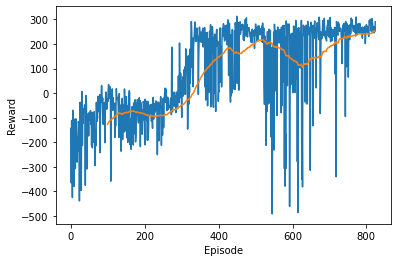env = gym.make('LunarLander-v2')
agent.policy_net.eval()
observation = env.reset()
render = False
rewards = []

for e in range(100):
cur_observation = env.reset()
if render:
env.render()
episode_reward = 0
for t in count():
action = agent.select_action(cur_observation)
next_observation, reward, done, info = env.step(action)
cur_observation = next_observation
episode_reward += reward
if render:
env.render()
if done:
rewards.append(episode_reward)
plot_rewards(rewards)
plt.pause(0.01)
print(f'Episode {e}: {episode_reward}')
if is_ipython:
display.clear_output(wait=True)
break

env.close()

plt.figure(2)
plt.clf()
plt.xlabel('Episode')
plt.ylabel('Reward')
plt.plot(rewards)
plt.axhline(y=np.mean(rewards), color='r', linestyle='-')
plt.savefig('out\\agent_performance.png')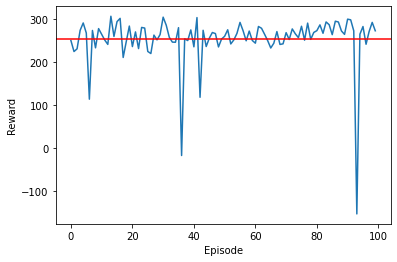# Hyper-Parameter Tuning

alphas = [0.01, 0.005, 0.001, 0.0005, 0.0001]
rewards_dict = {}

for a in alphas:
run_rewards, agent = lander_runner(
num_episodes=1500,
target_update=10,
alpha=a,
eps=1,
eps_decay=0.99,
gamma=0.999,
seed=42
)
rewards_dict[a] = moving_average(run_rewards, 100)
plot_multiple_rewards('\u03B1', rewards_dict)
plt.savefig('out\\alpha_learning_curve.png')

Solved in 1063 episodes.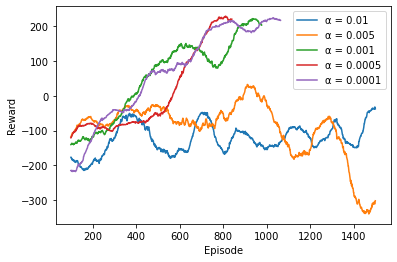gamma = [0.9, 0.99, 0.995, 0.999]
rewards_dict = {}

for g in gamma:
run_rewards, agent = lander_runner(
num_episodes=1500,
target_update=10,
alpha=0.0005,
eps=1,
eps_decay=0.99,
gamma=g,
seed=42
)
rewards_dict[g] = moving_average(run_rewards, 100)
plot_multiple_rewards('\u03B3', rewards_dict)
plt.savefig('out\\gamma_learning_curve.png')

Solved in 835 episodes.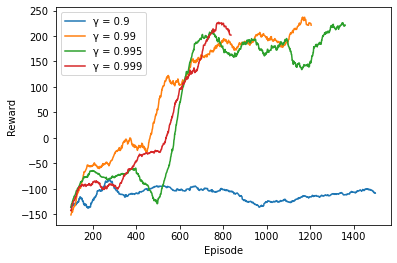target_update = [2, 4, 8, 10]
rewards_dict = {}

for tu in target_update:
run_rewards, agent = lander_runner(
num_episodes=1500,
target_update=tu,
alpha=0.0005,
eps=1,
eps_decay=0.99,
gamma=0.999,
seed=42
)
rewards_dict[tu] = moving_average(run_rewards, 100)
plot_multiple_rewards('Target Network Update', rewards_dict)
plt.savefig('out\\target_update_learning_curve.png')

Solved in 935 episodes.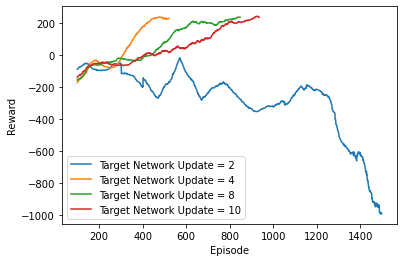# The impact of the second network

To see the impact the second network makes, we run the experiment with the SimpleDQNAgent. Here we see the agent is unable to learn anything useful, never breaking above 0 points, meaning it is always crashing. It terminates after 1500 episodes.

def simple_lander_runner(num_episodes, target_update, alpha, eps, eps_decay, gamma, seed, render=False):
env = gym.make('LunarLander-v2')
env.seed(seed)
agent = SimpleDQNAgent(env.observation_space.shape, env.action_space.n,
alpha=alpha, eps=eps, eps_decay=eps_decay, gamma=gamma, seed=seed)

rewards = []

for e in range(num_episodes):
cur_observation = env.reset()
if render:
env.render()
episode_reward = 0
for t in count():
action = agent.select_action(cur_observation)
next_observation, reward, done, info = env.step(action)
agent.update_q(cur_observation, action, next_observation, reward, done)
cur_observation = next_observation
episode_reward += reward
if render:
env.render()
if done:
rewards.append(episode_reward)
plot_rewards(rewards)
plt.pause(0.01)
print(f'Episode {e}: {episode_reward}')
if is_ipython:
display.clear_output(wait=True)
break
if np.all(moving_average(rewards, 100)[-100:] >= 200):
print(f'Solved in {e} episodes.')
agent.save_network(f'out\\agent.pt')
break

env.close()
return rewards, agent

run_rewards, agent = simple_lander_runner(
num_episodes=1500,
target_update=4,
alpha=0.0005,
eps=1,
eps_decay=0.99,
gamma=0.999,
seed=57
)
plot_rewards(run_rewards)
plt.savefig('out\\simple_learning_curve.png')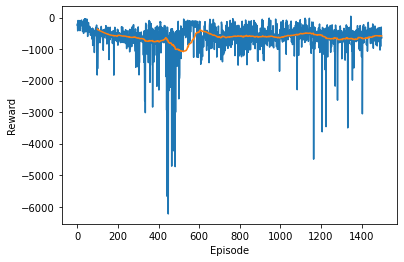1. Mnih, V., Kavukcuoglu, K., Silver, D., Graves, A., Antonoglou, I., Wierstra, D., & Riedmiller, M. (2013). Playing Atari with Deep Reinforcement Learning. https://arxiv.org/abs/1312.5602